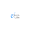## What is the full form of CPTL?

• Complimentary Pass Transistor Logic

### What does CPTL mean?

The complexity of the full CMOS pass gate logic circuit can be dramatically reduced by using a different circuit concept known as CPTL. The main idea behind CPL is to perform logic operations on a purely NMOS pass transistor network rather than a CMOS TG network. All inputs are applied in complementary form, which means that each input signal and its signal and its inverse must be provided, the circuit also generates compumetray outputs. Which is used by subsequent CPL stages. As a result, the CPL circuit consists primarily of complementary input, an NMOS pass transistor logic network to generate complementary outputs, and CMOS output inverters to restore output signals.

## What is the full form of CPTL?

• Complimentary Pass Transistor Logic

### What does CPTL mean?

The complexity of the full CMOS pass gate logic circuit can be dramatically reduced by using a different circuit concept known as CPTL. The main idea behind CPL is to perform logic operations on a purely NMOS pass transistor network rather than a CMOS TG network. All inputs are applied in complementary form, which means that each input signal and its signal and its inverse must be provided, the circuit also generates compumetray outputs. Which is used by subsequent CPL stages. As a result, the CPL circuit consists primarily of complementary input, an NMOS pass transistor logic network to generate complementary outputs, and CMOS output inverters to restore output signals.

1.